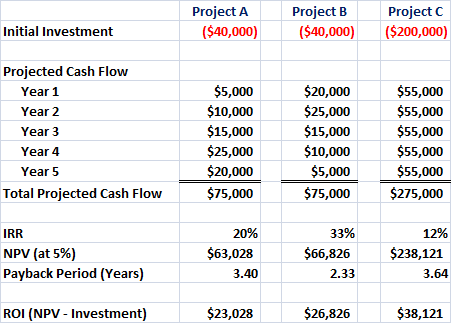# Discounted Payback Period Method Calculation with Examples

Post on: 16 Март, 2015Posted by financialmemos on November 23, 2013

The discounted payback period method is exactly the same as that simple payback period method (which was explained in a different  post ) apart from one thing. The discounted payback period method accounts for the time value of money. In other words, it allows us to discount the future cash inflows (or outflows) and calculate the present the value of them.

By doing that, we can calculate the payback period or the time that it takes for a project to break even by correctly accounting for the fact that having \$1 now does not have the same value as having \$1 in 5 years from now.

## Discounted Payback Period Formula & Calculation

As explained above, the discounted payback period is used to calculate the time that it takes for a project to bring in enough profits to cover the initial investment (and other subsequent costs) or put it differently, how many years it takes to break even and recoup the initial investment.

Trying to give a single formula will complicate things but an example will help us understand how the payback period is calculated. Lets assume that company ABC has the option to invest in a project that will initially cost \$20,000. The project will run for 4 years and will bring the following profits during each one of these four years.

Year 1 =  \$7,000

Year 2=  \$9,000

Year 3=  \$4,000

Year 4= \$12,000

The company believes that the cost of capital that should be used to discount these cash flows is 10% since thats the interest rate the bank charges Company ABC for the loan facility.

Company ABC uses the discounted payback period method (among other methods) to rank potential projects and choose the ones that will be undertaken.

## Discounted Payback Period Example

Using the example explained above, we will need to perform the following steps to calculate the discounted payback period.

-First Step. Calculate the discounted cash flow for each period by using the following formula :

Discounted Cash flows =  (Cash flows)/ (1+r)^n

where r is the cost of capital or 10% and n is the time (for this example we have 4 years so n spans from zero to four).

-Second Step: Calculate the cumulative discounted cash flows until we get a cumulative cash flow number that is greater than zero

-Third Step: When we get the first positive number we know that the project has started bringing profits so the discounted payback period can be now calculated. What we need to do is workout how many months from this last period we need to add in order for the project to make zero profit (neither a loss nor a profit).

Lets see the above steps by using the example already provided.

More from this section
Categories
Tags

• Most Popular